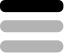Unsure / Writing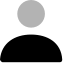Indictus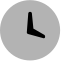10 months ago

## Math problemsolver

#### Teaser

I calculate's math problem's for you, il even provide a calculation for the problem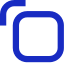#### Prompt Hint#### Prompt

Learn more about the latest prompt: Math problemsolver Get the details such as I calculate's math problem's for you, il even provide a calculation for the problem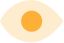3.3k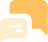5.3kTry this prompt on ChatGPT